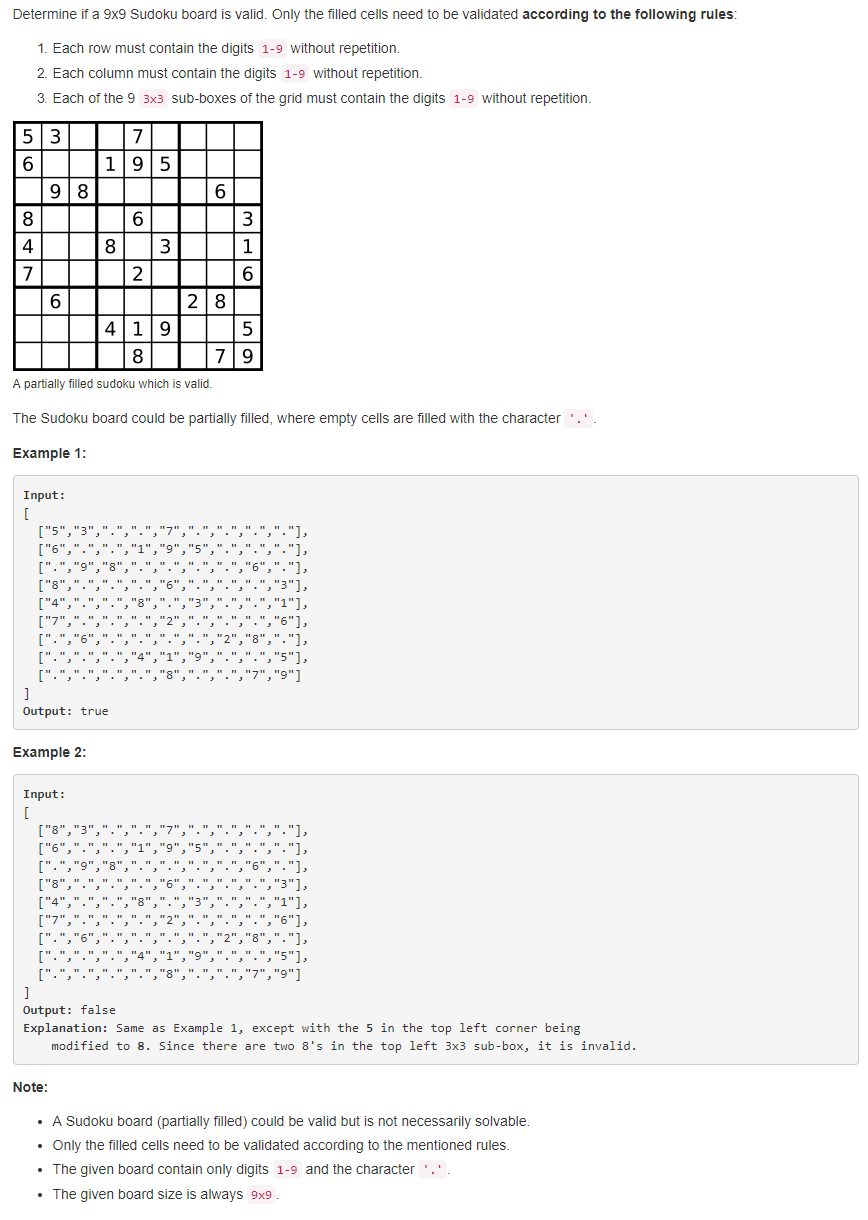# 题目描述（中等难度）• 每一行的数字不能重复

• 每一列的数字不能重复

• 9 个 3 * 3 的小棋盘中的数字也不能重复。

# 解法一 暴力解法

public boolean isValidSudoku(char[][] board) {
//判断每一行
for (int i = 0; i < 9; i++) {
if (!isValidRows(board[i])) {
return false;
}
}
//判断每一列
for (int i = 0; i < 9; i++) {
if (!isValidCols(i, board)) {
return false;
}
}
//判断每个小棋盘
for (int i = 0; i < 9; i = i + 3) {
for (int j = 0; j < 9; j = j + 3) {
if (!isValidSmall(i, j, board)) {
return false;
}
}

}
return true;
}

public boolean isValidRows(char[] board) {
HashMap<Character, Integer> hashMap = new HashMap<>();
for (char c : board) {
if (c != '.') {
if (hashMap.getOrDefault(c, 0) != 0) {
return false;
} else {
hashMap.put(c, 1);
}
}
}
return true;
}

public boolean isValidCols(int col, char[][] board) {
HashMap<Character, Integer> hashMap = new HashMap<>();
for (int i = 0; i < 9; i++) {
char c = board[i][col];
if (c != '.') {
if (hashMap.getOrDefault(c, 0) != 0) {
return false;
} else {
hashMap.put(c, 1);
}
}
}
return true;
}

public boolean isValidSmall(int row, int col, char[][] board) {
HashMap<Character, Integer> hashMap = new HashMap<>();
for (int i = 0; i < 3; i++) {
for (int j = 0; j < 3; j++) {
char c = board[row + i][col + j];
if (c != '.') {
if (hashMap.getOrDefault(c, 0) != 0) {
return false;
} else {
hashMap.put(c, 1);
}
}
}
}
return true;
}


# 解法二

• 如果第 4 行有一个数字 8，我们就 (8)4，把 "(8)4"放进去。
• 如果第 5 行有一个数字 6，我们就 5(6)，把 "5(6)"放进去。
• 小棋盘看成一个整体，总共是 9 个，3 行 3 列，如果第 2 行第 1 列的小棋盘里有个数字 3，我们就把 "2(3)1" 放进去。

public boolean isValidSudoku(char[][] board) {
Set seen = new HashSet();
for (int i=0; i<9; ++i) {
for (int j=0; j<9; ++j) {
if (board[i][j] != '.') {
String b = "(" + board[i][j] + ")";
return false;
}
}
}
return true;
}


public boolean isValidSudoku(char[][] board) {
Set seen = new HashSet();
for (int i=0; i<9; ++i) {
for (int j=0; j<9; ++j) {
char number = board[i][j];
if (number != '.')
if (!seen.add(number + " in row " + i) ||
!seen.add(number + " in column " + j) ||
!seen.add(number + " in block " + i/3 + "-" + j/3))
return false;
}
}
return true;
}